# Function Table Worksheets

#### What are Function Tables?

They express the inputs and similar outputs of a function. You can make these tables in vertical and horizontal (up down or side to side) pattern. In case of horizontal style of tables, there are two rows in which one expresses the similar outputs and the other one shows the input. You can also make this table with these details. For instance, you can track the cost of candies with their total number of bars. You need to put all of this information into the table. You will know that the calculated total cost of candies. If you have total 10 candies so, the total price of all candies will be 20 dollars. Each function that you exercise in the table explain the relationship between input and the outputs. You have two options of using function tables. You can use it by describing input and the similar outputs in words or by using an equation. First option is the best way of using function table.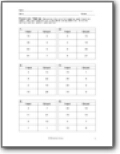###### What's the Rule?

Determine the rule followed by each function table. The rule consists of 2 variables using addition, division, multiplication, and/or subtraction.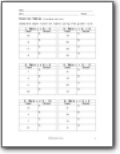###### 2 Variables-Input Only

Complete each function table using the given rule.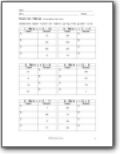###### Missing Inputs and Outputs

Complete each table as it is missing an input or output.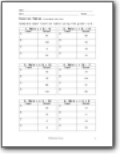###### Double Operators

Each equation has two operators involved.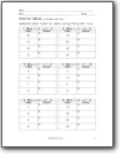###### Triple Operations

These inputs get pushed through three different operations.